Next: Affine Reconstruction Up: 3D Reconstruction from Multiple Previous: 3D Reconstruction from Multiple

## Projective Reconstruction

Simple parameter counting shows that we have 2nm independent measurements and only 11 m + 3 n unknowns, so with enough points and images the problem ought to be soluble. However, the solution can never be unique as we always have the freedom to change the 3D coordinate system we use. In fact, in homogeneous coordinates the equations become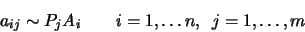(5.4)

So we always have the freedom to apply a nonsingular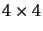transformation H to both projections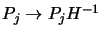and world points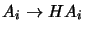. Hence, without further constraints, reconstruction is only ever possible up to an unknown projective deformation of the 3D world. However, modulo this fundamental ambiguity, the solution is in general unique.

One simple way to obtain the solution is to work in a projective basis tied to the 3D points . Five of the visible points (no four of them coplanar) can be selected for this purpose.

Exercise 5.1   : Given the epipolar geometry, show how we can decide whether four points are coplanar or not, by just considering their images in a stereo pair. Hint: consider the intersection of a pair of image lines, each linking two of the four points.

An alternative to this is to select the projection center of the first camera as the coordinate origin, the projection center of the second camera as the unit point, and complete the basis with three other visible 3D points A1, A2, A3 such that no four of the five points are coplanar.

Exercise 5.2   : Design an image-based test to check whether three points are coplanar with the center of projection. Derive a test that checks that two points are not coplanar with the base line of a stereo pair, assuming that the epipolar geometry is known. Deduce a straightforward test to check that the above five points form a valid 3D projective basis.

Let a1, a2, a3 and a'1, a'2, a'3 respectively be the projections in image 1 and image 2 of the 3D points A1, A2, A3. Make a projective transformation of each image so that these three points and the epipoles become a standard basis: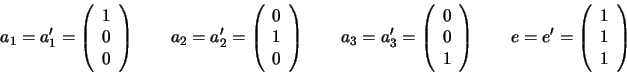Also fix the 3D coordinates of A1, A2, A3 to be respectively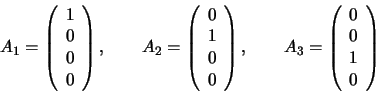It follows that the two projection matrices can be written: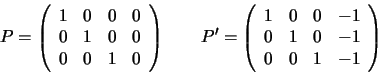Exercise 5.3   : Show that the projections have these forms. (NB: Given only the projections of A1,A2,A3, each row of P,P' could have a different scale factor since point projections are only defined up to scale. It is the projections of the epipoles that fix these scale factors to be equal).

Since the projection matrices are now known, 3D reconstruction is relatively straightforward. This is just a simple, tutorial example so we will not bother to work out the details. In any case, for precise results, a least squares fit has to be obtained starting from this initial algebraic solution (e.g. by bundle adjustment).Next: Affine Reconstruction Up: 3D Reconstruction from Multiple Previous: 3D Reconstruction from Multiple
Bill Triggs
1998-11-13